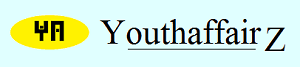June 2012

# Solution

The ages of the three children are 3, 3 and 8 years.

The product of the ages of the three children is 72 and the sum of the ages is the same as the house number.

Therefore, considering the factors of 72, the list of possibilities is as follows:

a)1, 1, 72 and the sum is 74

b)1, 2, 36 and the sum is 39

c)1, 3, 24 and the sum is 28

d)1, 4, 18 and the sum is 23

e)1, 6, 12 and the sum is 19

f)1, 8, 9 and the sum is 18

g)2, 2, 18 and the sum is 22

h)2, 3, 12 and the sum is 17

i)2, 4, 9 and the sum is 15

j)2, 6, 6 and the sum is 14

k)3, 3, 8 and the sum is 14

l)3, 4, 6 and the sum is 13

Ajit has prepared this list. Now all that he has to do is look at the house number and he would be able to tell the ages. For example, if the house number is 19, then option (e) will apply and the ages of the children will be 1, 6 and 12. Likewise, if the house number is 18, then option (f) will appply and the ages of the children will be 1, 8 and 9. Every sum is unique so it would be easy to find the ages based on the house number. But, Ajit cannot figure out the ages still although he is good at mathematics. This means that the house number is such that it occurs in more than one option. There are two options - (j) and (k), both of which have the same sum 14. The house number, therefore, is definitely 14 and Ajit cannot figure out whether the ages of the children are 2, 6 and 6 or 3, 3 and 8. So he asks for a further hint.

The hint given is: "The oldest child likes adventure sports." It is not the sport which matters, but now Ajit knows for sure that there is an oldest child. In case of option (j), the ages are 2, 6 and 6. That is there are are two children of the same age, six. There is, thus, no single eldest child. This, therefore, cannot be the answer. So the only possiblity is option (k) where there is a single oldest child aged 8 and twins who are three years old.

• Union Public Service Commission - www.upsc.gov.in
• IIT-Kharagpur - www.iitkgp.ac.in
• Indian Statistical Institute - www.isical.ac.in
• Indian Institute of Technology Madras - www.iitm.ac.in
• Indian Institute of Management, Ahmedabad - www.iimahd.ernet.in
• Indian Institute of Mass Commission - www.iimc.nic.in
• IIT Bombay - www.iitb.ac.in